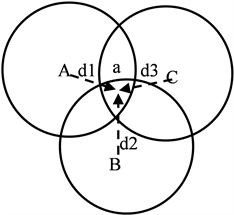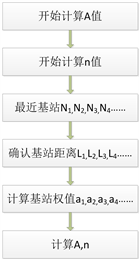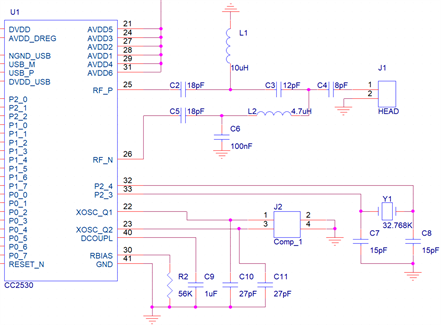﻿ 基于ZigBee的关键区域人员定位技术研究

# 基于ZigBee的关键区域人员定位技术研究Research on Personnel Location Technology in Key Areas Based on ZigBee

Abstract: With the rapid development of social economy, agencies, large enterprises and safety management units are paying more attention to the realization of personnel positioning and trajectory manage-ment. Managers need to accurately grasp the actual location, entry and exit, and residence time of personnel to improve business management and emergency management efficiency. In order to achieve the problem of refined personnel management, this paper chooses to use ZigBee technology and RSSI weighted positioning algorithm for precise personnel positioning, providing an effective solution for relevant units to help relevant units grasp the real-time distribution of on-site person-nel in the production area and achieve early warning of personnel risks in areas with major haz-ards.

1. 引言

2. 无线定位机制Figure 1. Triangulation

$\left\{\begin{array}{l}d1=\frac{T1a-T}{2}*V\text{\hspace{0.17em}}\text{\hspace{0.17em}}\text{\hspace{0.17em}}\text{\hspace{0.17em}}\text{\hspace{0.17em}}\text{\hspace{0.17em}}\text{\hspace{0.17em}}\text{\hspace{0.17em}}\text{\hspace{0.17em}}\text{\hspace{0.17em}}\text{\hspace{0.17em}}\text{\hspace{0.17em}}\text{\hspace{0.17em}}\left(2\text{-}1\right)\\ d2=\frac{T1b-T}{2}*V\text{\hspace{0.17em}}\text{\hspace{0.17em}}\text{\hspace{0.17em}}\text{\hspace{0.17em}}\text{\hspace{0.17em}}\text{\hspace{0.17em}}\text{\hspace{0.17em}}\text{\hspace{0.17em}}\text{\hspace{0.17em}}\text{\hspace{0.17em}}\text{\hspace{0.17em}}\text{\hspace{0.17em}}\text{\hspace{0.17em}}\left(2\text{-2}\right)\\ d3=\frac{T1c-T}{2}*V\text{\hspace{0.17em}}\text{\hspace{0.17em}}\text{\hspace{0.17em}}\text{\hspace{0.17em}}\text{\hspace{0.17em}}\text{\hspace{0.17em}}\text{\hspace{0.17em}}\text{\hspace{0.17em}}\text{\hspace{0.17em}}\text{\hspace{0.17em}}\text{\hspace{0.17em}}\text{\hspace{0.17em}}\text{\hspace{0.17em}}\text{\hspace{0.17em}}\left( 2 -3 \right)\end{array}$

$\left\{\begin{array}{l}RSSI=-\left(10n\mathrm{lg}d+A\right)\text{\hspace{0.17em}}\text{\hspace{0.17em}}\text{\hspace{0.17em}}\text{\hspace{0.17em}}\text{\hspace{0.17em}}\text{\hspace{0.17em}}\text{\hspace{0.17em}}\text{\hspace{0.17em}}\text{\hspace{0.17em}}\text{\hspace{0.17em}}\text{\hspace{0.17em}}\text{\hspace{0.17em}}\text{\hspace{0.17em}}\text{\hspace{0.17em}}\text{\hspace{0.17em}}\text{\hspace{0.17em}}\text{\hspace{0.17em}}\text{\hspace{0.17em}}\text{\hspace{0.17em}}\text{\hspace{0.17em}}\text{\hspace{0.17em}}\left(3\text{-}1\right)\\ P\left(d\right)=P\left({d}_{0}\right)+10n\mathrm{lg}\left(\frac{1}{2}\right)+{\mathcal{l}}_{\partial }\text{\hspace{0.17em}}\text{\hspace{0.17em}}\text{\hspace{0.17em}}\text{\hspace{0.17em}}\text{\hspace{0.17em}}\text{\hspace{0.17em}}\text{\hspace{0.17em}}\text{\hspace{0.17em}}\text{\hspace{0.17em}}\text{\hspace{0.17em}}\left( 3 -2 \right)\end{array}$

$n=\frac{\underset{i=1}{\overset{w}{\sum }}{a}_{i}×{n}_{i}}{\underset{i=1}{\overset{w}{\sum }}{a}_{i}}$ (4-1)Figure 2. Weighted RSSI algorithm flowchart

5. 系统设计方案Figure 3. CC2530 Peripheral circuitsTable 1. Coordinate comparison between actual position and measured position

6. 结论

 刘勇. 基于ZigBee的无线网络传感器定位技术[J]. 计算机产品与流通, 2020(10): 104.

 胡建芳, 胡志军, 李利飞. 无线传感器网络定位技术研究[J]. 信息与电脑(理论版), 2018(24): 146-148.

 石欣, 印爱民, 陈曦. 基于RSSI的多维标度室内定位算法[J]. 仪器仪表学报, 2014, 34(2): 36-40.

 高鹏, 石为人, 周伟, 等. 基于图论模糊聚类的室内自适应RSSI定位算法[J]. 仪器仪表学报, 2013, 34(9): 1998- 2003.

 刘飞飞, 徐隆姬, 马礼然. 基于ZigBee的分布式农业环境监测系统设计[J]. 传感器与微系统, 2021, 40(3): 90-92.

 李战明, 李振兴. ZigBee技术在人员搜救系统中的应用[J]. 电子测量与仪器学报, 2012, 25(5): 186-190.

 王战备. 基于ZigBee的农田信息监测网络设计[J]. 国外电子测量技术, 2013, 32(8): 42-45.

 吕宏, 黄钉劲. 基于ZigBee技术低功耗无线温度数据采集及传输[J]. 国外电子测量技术, 2012, 31(2): 58-60.

 王森. 基于物联网的多功能智能家居系统设计[J]. 电子世界, 2020, 66(5): 122-123+126.

 周洪伟, 罗建, 王韫, 等. 外场环境信息无线监测系统设计[J]. 国外电子测量技术, 2011, 30(4): 66-68.

Top